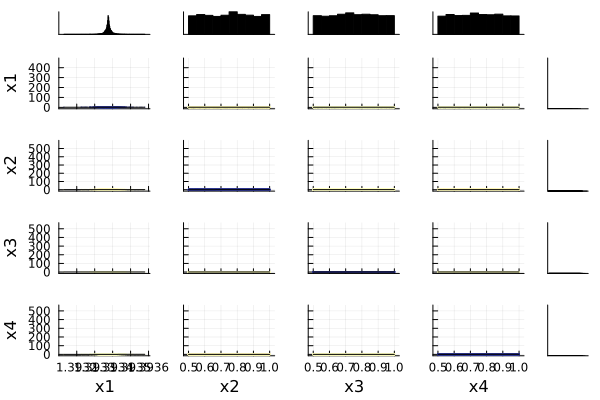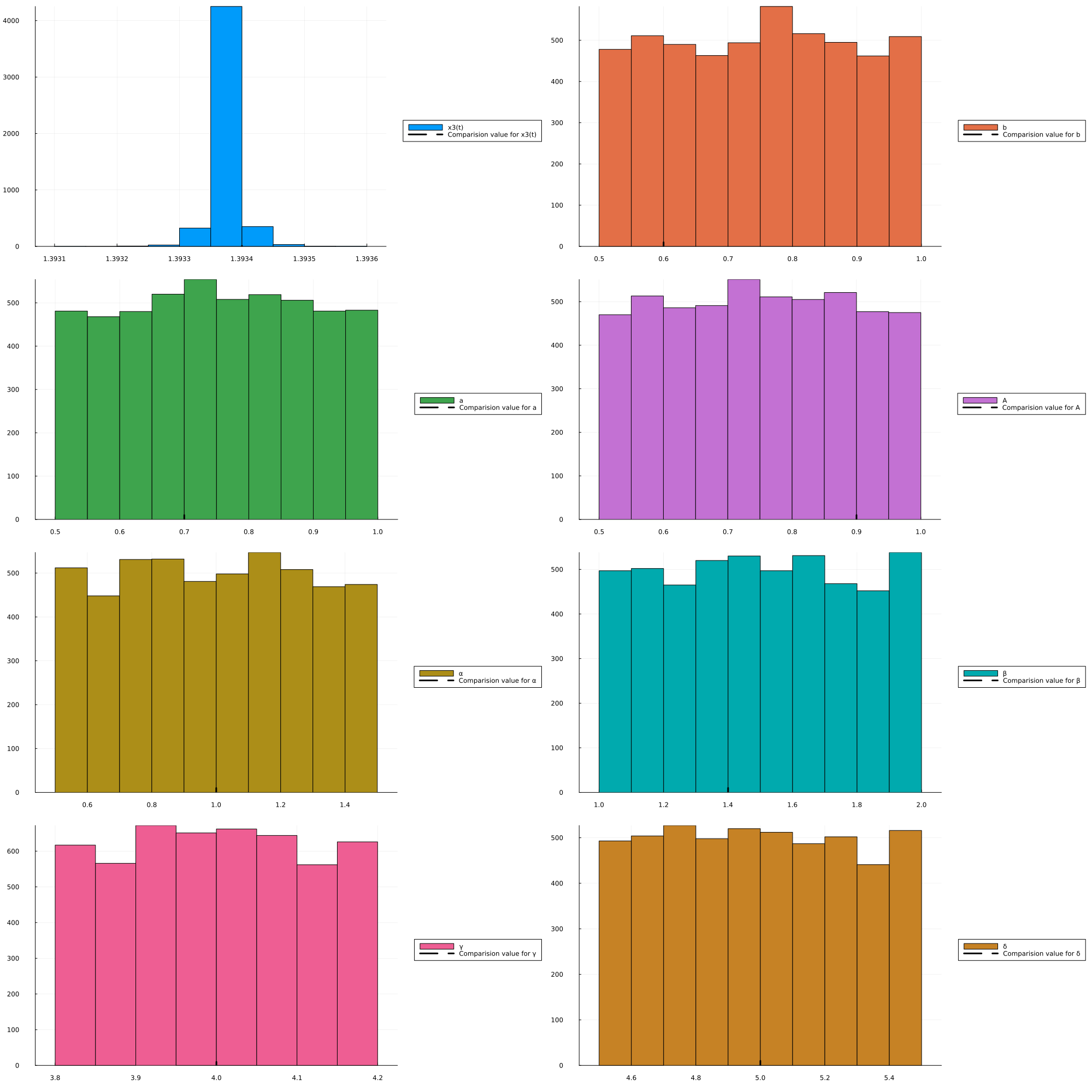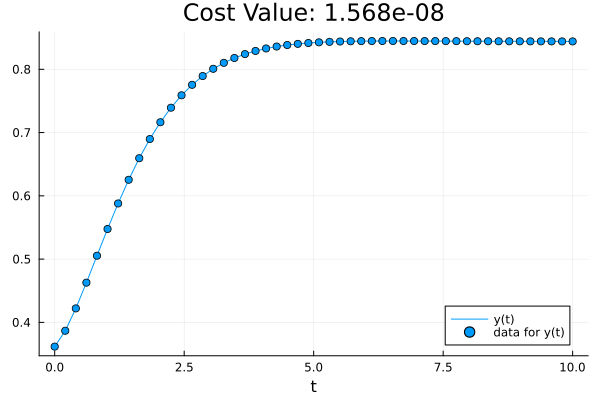# Structural and Practical Identifiability Analysis of Goodwin Enzymetic Oscillator

In this tutorial we will show a classical model of oscillations in enzyme kinetics proposed by Goodwin in 1965 (,). It is a good-benchmarking example for comparing the performance of several structural identifiability methods, considering two different scenarios: when the three states are measured, or when only one of them, i.e. the enzyme concentration, that can be measured. This is a system of equations, as shown below, contains 3 states, 1 observable and 8 unknown parameters as shown below:

\begin{aligned} \dot x_1 &= -b x_1 + \frac{a}{A+x_3} \\ \dot x_2 &= \alpha x_1 - \beta x_2 \\ \dot x_3 &= \gamma x_2 - \delta x_3 \end{aligned}

## Julia Environment

For this tutorial we will need the following packages:

ModuleDescription
PumasQSPPumasQSP is used to formulate our problem and perform automated model discovery
ModelingToolkitThe symbolic modeling environment
DifferentialEquationsThe numerical differential equation solvers
DataSetsWe will load our experimental data from datasets on JuliaHub
DataFramesHandling tabular data manipulation
StructuralIdentifiabilityAssessing structural parameter identifiability of parametric ODE models
StatsPlotsThe plotting and visualization library

Besides that we'll also need the following Julia standard library:

ModuleDescription
RandomRequired to set seed

First we prepare the environment by listing the packages we are using within the example.

#Packages used for this example
using PumasQSP
using ModelingToolkit
using DifferentialEquations
using DataSets
using DataFrames
using StructuralIdentifiability: assess_identifiability, assess_local_identifiability
using Random
using StatsPlots

## Model Set-up and Assessing Structural Identifiability

We will start our analysis with by using structural identifiability analysis tooling. Structural identifiability analysis is symbolic and assumes perfect measurement. It calculates whether it's even mathematically possible to identify given parameters with the chosen observables even when there is infinite data on the observables. While a good starting point for understanding parametric identifiability, this method is unable to say whether the true amount of data is sufficient for practically identifying the parameters from data (i.e. it does not do practical identifiability analysis). Also, such a symbolic method is difficult to scale to high dimensions, and thus is only possible on small models such as one demonstrated here.

To perform structural identifiability analysis, we will use assess_local_identifiability from StructuralIdentifiability.jl as follows:

iv = @variables t
states = @variables x1(t) = 0.3617 x2(t) = 0.9137 x3(t) = 1.3934
@variables y(t)
ps = @parameters b=0.5 a=0.5 A=0.8 α=0.8 β=1.2 γ=3.8 δ=4.5
D = Differential(t)

eqs = [
D(x1) ~ -b*x1 + (a/(A + x3)),
D(x2) ~ α*x1 - β*x2,
D(x3) ~ γ*x2 - δ*x3
]

obs_eq = [y ~ x1]
measured_quantities = [y ~ x1]

@named model = ODESystem(eqs, t, states, ps; observed = obs_eq)

# Local Identifiability Check
assess_local_identifiability(model, measured_quantities = measured_quantities,p = 0.99)
Dict{SymbolicUtils.BasicSymbolic{Real}, Bool} with 10 entries:
a     => 0
α     => 0
β     => 1
δ     => 1
γ     => 0
x1(t) => 1
x3(t) => 0
b     => 1
A     => 0
x2(t) => 0

From above observations we can see that not all of the parameters are found to be locally identifiabile, and this is validated from the un-identifiable peaks for each of the parameters, that is described by the histogram plots below. We can also run a global identifiable check as defined below, where it will first run the local identifiable checks, and then compute the Wronskians, to proceed for the global identifiability checks, which adds overheads to the overall compute time. Depending on the tolerance for the probability of accuracy with which the parameters can be identifiable, can also effect the compute-time taken to complete the identifiability check, for example changing the probability kwarg to p=0.9, can significantly reduce the run-time.

# Global Identifiability Check
assess_identifiability(model, measured_quantities = measured_quantities,p = 0.99)
Dict{SymbolicUtils.BasicSymbolic{Real}, Symbol} with 7 entries:
a => :nonidentifiable
α => :nonidentifiable
β => :locally
δ => :locally
γ => :nonidentifiable
b => :globally
A => :nonidentifiable

## Analyzing Practical Identifiability via Virtual Populations

Knowing that the model is structurally identifiable means that with noise-less infinite data on the chosen observables, the parameter values are expected to be exactly identifiable. However, in practice our data has noise and is not infinite. Thus we wish to understand how identifiable our parameters really are given the data we have on hand. This is one way to define practical identifiability analysis. While there are many ways to perform a practical identifiability analysis, one simple way is to use virtual populations to find an ensemble of fitting parameters and analyzing the resulting distribution of parameters which all equally fit the data. We will demonstrate this using Pumas-QSP's vpops functionality.

First we define our trial. This is done as follows:

trial_inits = [x1 => 0.3617,
x2 => 0.9137]

trial_ps = [b => 0.6,
a => 0.7,
A => 0.9,
α => 1,
β => 1.4,
γ => 4,
δ => 5
]

Next we import our trial data.

Goodwin_oscillator_data = dataset("pumasqsptutorials/Goodwin_oscillator_data")

trial = Trial(Goodwin_oscillator_data, model;
u0= trial_inits,
params= trial_ps ,  save_names = [y],
tspan=trial_tspan)
Trial with parameters:
b => 0.6
a => 0.7
A => 0.9
α => 1.0
β => 1.4
γ => 4.0
δ => 5.0 and initial conditions:
x1(t) => 0.3617
x2(t) => 0.9137.
timespan: (0.0, 10.0)


## Creating the inverse problem

After we have built our experiment, the next step is to define the inverse problem, i.e. specifying the parameters that we want to optimize and the search space in which we want to look for them. The inverse problem consists of a collection of experiments which define a multi-simulation optimization problem. The solution to an inverse problem are the "good parameters" which make the simulations simultaneously fit their respective data sufficiently well. In this context, we have only created a single experiment.

Random.seed!(1234)
prob = InverseProblem(trial, model, [
x3  => (1.0,2),
b => (0.5,1),
a => (0.5,1),
A => (0.5,1),
α => (0.5,1.5),
β => (1,2),
γ => (3.8,4.2),
δ => (4.5,5.5)
])

vp = vpop(prob, StochGlobalOpt(maxiters = 2_000), population_size = 5_000)
Virtual population of size 5000 computed in 24 minutes and 34 seconds.
┌─────────┬──────────┬──────────┬──────────┬──────────┬─────────┬─────────┬─────
│   x3(t) │        b │        a │        A │        α │       β │       γ │    ⋯
├─────────┼──────────┼──────────┼──────────┼──────────┼─────────┼─────────┼─────
│ 1.39337 │ 0.885512 │ 0.549346 │ 0.623675 │ 0.638886 │ 1.75916 │ 3.87757 │ 4. ⋯
│ 1.39337 │  0.68704 │ 0.686627 │ 0.711661 │ 0.647494 │ 1.05767 │ 3.93424 │  4 ⋯
│ 1.39338 │ 0.757478 │ 0.672856 │ 0.826435 │ 0.863743 │ 1.58708 │ 4.12219 │ 5. ⋯
│ 1.39341 │ 0.618875 │ 0.530156 │ 0.500007 │  1.43675 │ 1.08732 │ 4.04735 │ 4. ⋯
│ 1.39337 │ 0.668122 │ 0.942921 │ 0.636018 │ 0.792846 │ 1.73153 │ 3.86123 │ 5. ⋯
│ 1.39338 │ 0.914912 │  0.78285 │ 0.670976 │ 0.825179 │ 1.61852 │ 4.17497 │ 5. ⋯
│ 1.39339 │ 0.600304 │ 0.502271 │ 0.737051 │  1.38796 │ 1.53039 │ 4.16575 │ 4. ⋯
│ 1.39338 │ 0.834233 │ 0.794008 │ 0.601441 │ 0.688278 │ 1.28879 │ 4.15595 │ 5. ⋯
│ 1.39338 │ 0.541162 │ 0.913655 │  0.91319 │  1.45044 │ 1.97887 │ 3.89963 │ 5. ⋯
│ 1.39337 │ 0.761292 │ 0.734532 │ 0.721595 │ 0.711432 │ 1.90624 │ 4.07687 │ 4. ⋯
│ 1.39337 │ 0.580165 │ 0.864026 │ 0.805925 │  1.46707 │ 1.92201 │ 4.10539 │ 4. ⋯
│ 1.39338 │ 0.579081 │  0.66301 │ 0.871905 │  1.28066 │ 1.92822 │ 4.17219 │ 4. ⋯
│ 1.39341 │ 0.506555 │ 0.788211 │ 0.727764 │  1.39068 │ 1.20046 │ 3.88838 │ 4. ⋯
│ 1.39339 │ 0.906291 │ 0.646874 │ 0.912727 │  1.22814 │ 1.02717 │ 3.90961 │ 4. ⋯
│ 1.39336 │ 0.561368 │ 0.999102 │ 0.801294 │  1.17352 │ 1.48871 │ 4.18542 │ 5. ⋯
│ 1.39338 │ 0.893152 │ 0.993168 │  0.89973 │  1.26411 │ 1.67839 │ 3.94678 │ 5. ⋯
│    ⋮    │    ⋮     │    ⋮     │    ⋮     │    ⋮     │    ⋮    │    ⋮    │    ⋱
└─────────┴──────────┴──────────┴──────────┴──────────┴─────────┴─────────┴─────
1 column and 4984 rows omitted


## Visualising the correlation manifold between the parameters

cornerplot(Matrix(DataFrame(vp)[:, 1:4]))## Validating the identifiability observations from StructuralIdentifiablity.jl

plot(vp, layout=(4,2), show_comparision_value = [1.3934, 0.6, 0.7, 0.9, 1, 1.4, 4, 5], max_freq = [10, 10, 10, 10, 10, 10, 10, 10], legend = :outerright, size = (2000, 2000))From the generated histogram plot observations, we can justify that not all of the parameters have peaks that can be uniquely identifiable. Hence, we can understand that it represents a practically un-identifiable system. We can notice a few local peaks, which may occur due to practical identifiability as we lack sufficient data, which arises due to sparse-sampling constraints. Let us look at the data fit using the generated vpop members below.

## Visualizing the accuracy in data fit out of the vpop members

confidenceplot(trial,vp,confidence=0,legend=true)As we can clearly see that the degree of cost in the data-fit looks sufficiently reasonable for the worst level of confidence that refers to the worst case parameters that we can have among all the virtual population members. We can thus conclude that although some of the parameters are not identifiable, however, our model can identify the parameters reasonably well.